# NCERT Solutions for Class 12 Maths Chapter 6 Application of Derivatives Miscellaneous Exercise

These NCERT Solutions for Class 12 Maths Chapter 6 Application of Derivatives Miscellaneous Exercise Questions and Answers are prepared by our highly skilled subject experts.

## NCERT Solutions for Class 12 Maths Chapter 6 Application of Derivatives Miscellaneous ExerciseQuestion 1.
Using differentials, find the approximate value of each of the following.
a. $$\left(\frac{17}{81}\right)^{\frac{1}{4}}$$
b. $$\text { (33) }^{-\frac{1}{5}}$$
Solution: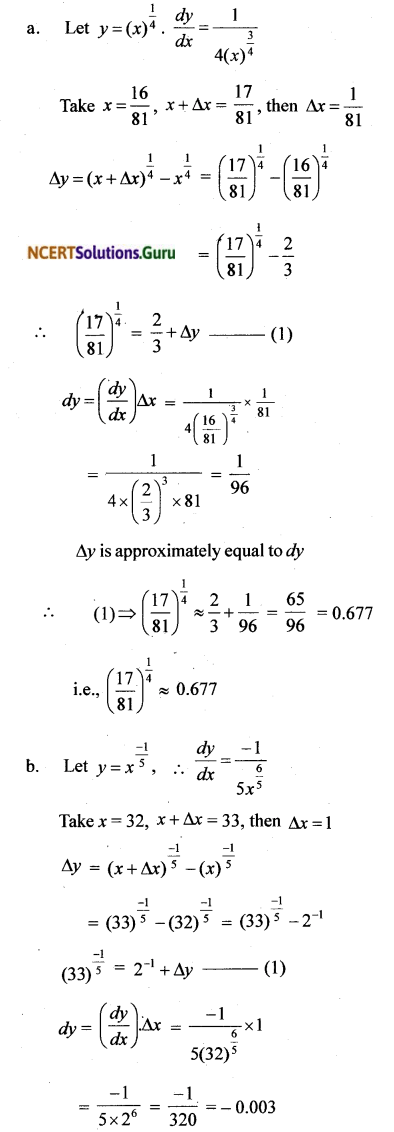∆y is approximately equal to dy
∴ (1) ⇒ (33)$$\frac { -1 }{ 5 }$$ ≈ 2-1 – 0.003
= 0.5 – 0.003 = 0.497
Hence (33)$$\frac { -1 }{ 5 }$$ ≈ 0.497

Question 2.
Show that the function given by f(x) = $$\frac { log x }{ x }$$ has maximum at x = e.
Solution: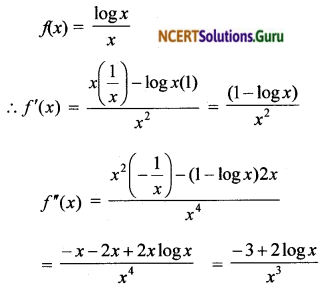For maxima, f'(x) = 0
i.e., $$\frac{1-\log x}{x^{2}}$$ = 0 ⇒ 1 – log x = 0
⇒ log x = 1 ⇒ x = e
At x = e, f”(e) = $$\frac{-3+2 \log e}{e^{3}}$$ < 0,
∴ f has a maximum at x = e

Question 3.
The two equal sides of an isosceles triangle with fixed base b are decreasing at the rate of 3 cm per second. How fast is the area decreasing when the two equal sides are equal to the base?
Solution:∆ ABC be an isosceles triangle with AB = AC
Let AB = x, BC = b, $$\frac { dx }{ dt }$$ = – 3cm/sec at
Draw AD ⊥ BC. Then D is the midpoint of BC.
i.e., BD = $$\frac { b }{ 2 }$$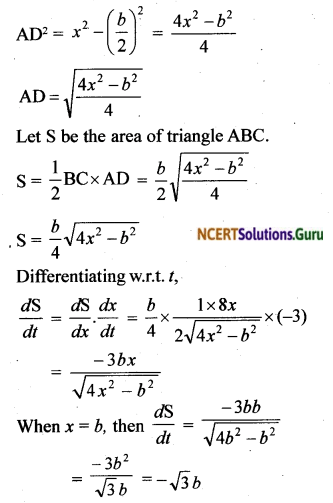Hence area is decreasing at the rate of $$\sqrt{3}$$ bcm²/sec.Question 4.
Find the equation of the normal to curve y² = 4x at the point (1,2).
Solution:
y² = 4x
Differentiating w.r.t. x,
2y$$\frac { dy }{ dx }$$ = 4
$$\frac { dy }{ dx }$$ = $$\frac { 2 }{ y }$$
At(1, 2), $$\frac { dy }{ dx }$$ = $$\frac { 2 }{ 2 }$$ = 1
Slope of the tangent at (1, 2) = $$\frac { -1 }{ 1 }$$ = – 1
Slope of the normal at(1, 2)
Equation of the tangent at (1, 2) is
y – 2 = 1(x – 1)
y – x – 1 = 0
Equation of the normal at (1, 2) is
y – 2 = 1(x – 1)
x + y – 3 = 0

Question 5.
Show that the normal at any point θ to the curve x = a cosθ + aθ sinθ, y = a sinθ – aθ cosθ is at a constant distance from the origin.
Solution:
x = acosθ + a0sinθ
y = asinθ – aθ cosθ
Differentiating w.r.t θ, dx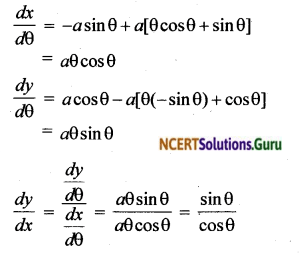Slope of the tangent at the point 0 is $$\frac { sinθ }{ cosθ }$$
∴ Slope of the normal at the point θ is $$\frac { – cosθ }{ sinθ }$$
∴ Equation of the normal at the point θ isi.e., xcosθ + ysin θ = a, which is the equation of the straight line in the normal form. Hence ‘a’ is the distance of the normal line from the origin. Thus the normal at any point 0 to the curve is at a constant distance from the origin.

Question 6.
Find the intervals in which the function f given by
f(x) = $$\frac{4 \sin x-2 x-x \cos x}{2+\cos x}, 0 \leq x \leq 2 \pi$$ (i) increasing (ii) decreasing.
Solution:Since f is continuous
i. f is increasing: 0 ≤ x ≤ $$\frac { π }{ 2 }$$ and $$\frac { 3π }{ 2 }$$ ≤ 2π
ii. is decreasing: $$\frac { π }{ 2 }$$ ≤ x ≤ $$\frac { 3π }{ 2 }$$Question 7.
Find the intervals in which the function f given by f(x) = x³ + $$\frac{1}{x^{3}}$$, x ≠ 0 is
i. increasing
ii. decreasing
Solution: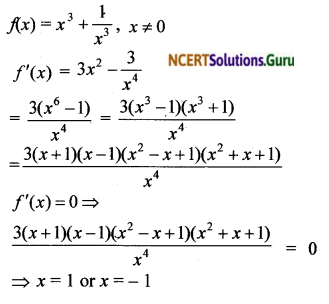The critical points are x = – 1, x = 0 and x =1. x = – 1, x = 0, x = 1 divide Rinto disjoint open intervals as(-∞, -1), (-1, 0),(0, 1) and (1, ∞)
i. f is increasing: x < – 1 and x > 1
ii. f is decreasing: – 1 < x < 0 and 0 < x < 1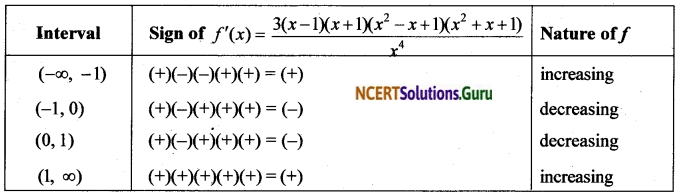Question 8.
Find the maximum area of an isosceles tri-angle inscribed in the ellipse $$\frac{x^{2}}{a^{2}}+\frac{y^{2}}{b^{2}}=1$$ = 1 with its vertex at one end of the major axis.
Solution: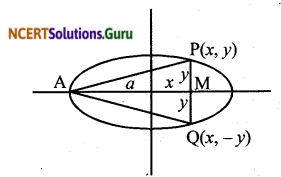Consider the ellipse $$\frac{x^{2}}{a^{2}}+\frac{y^{2}}{b^{2}}=1$$ = 1 , a > b
Let A be one end point of the major axis and P(x, y) be a point on the ellipse. Draw PM perpendicular to x axis and extend it to meet the ellipse at Q.
Then the coordinate of Q is (x, – y)
∆APQ is an isosceles triangle
Let S be the area of ∆APQ
S = $$\frac { 1 }{ 2 }$$PQ.AM = $$\frac { 1 }{ 2 }$$(2y)(x + a)
S = y(x + a) = $$\frac { b }{ 2 }$$$$\sqrt{a^{2}-x^{2}}$$(x + a)
Since S is positive, S² is maximum when S is maximumWhen x = – a, the point P coincides with A which is not possible.
∴ x = – $$\frac { a }{ 2 }$$
When x = $$\frac { a }{ 2 }$$, $$\frac{d^{2}}{d x^{2}}\left(\mathrm{~S}^{2}\right)$$
= $$\frac{-12 b^{2}}{a^{2}} \frac{a}{2}\left(\frac{a}{2}+a\right)$$ < 0
∴ S² is maximum when x = $$\frac { a }{ 2 }$$
i.e., S is maximum when x = $$\frac { a }{ 2 }$$
The maximum value of SQuestion 9.
A tank with rectangular base and rectangular sides, open at the top is to be constructed so that its depth is 2 m and volume is 8m³. If building of tank costs ₹ 70 per sq.metre for the base and ₹ 45 per square metre for sides, what is the cost of least expensive tank?
Solution:
Let x and y be the length and breadth of the base of the tank (in metre). Let C be the cost of building the tank.Volume of the tank = 8m³ (x)(y).(2) = 8 ⇒ xy = 4
∴ y = $$\frac { 4 }{ x }$$
Area of base = xy
Area of 4 sides = 2(x + y)(2) = 4(x + y)
Cost of constructionBut x = – 2 is not possible, since length of the base cannot be negative
∴ x = 2 When x = 2,
$$\frac{d^{2} \mathrm{C}}{d x^{2}}=\frac{180 \times 8}{8}$$ > 0
The minimum cost of construction,
C = 280 + 180(2 + $$\frac { 4 }{ 2 }$$) = ₹ 1000Question 10.
The sum of the perimeter of a circle and square is k, where k is some constant. Prove that the sum of their areas is least when the side of square is double the radius of the circle.
Solution:
Let r be the radius and x be the side of the square.
Then 2πr + 4x = k
∴ r = $$\frac{k-4 x}{2 \pi}$$ … (1)
Let S be the sum of areas of circle and square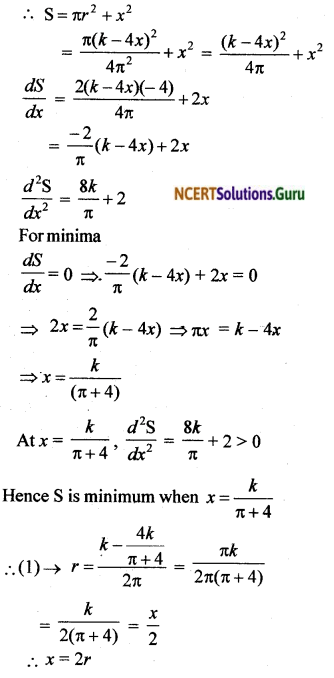Question 11.
A window is in the form of a rectangle sur-mounted by a semicircular opening. The total perimeter of the window is 10 m. Find the dimensions of the window to admit maximum light through the whole opening.
Solution:
Length of the window = 2x,
Height of the rectangular portion = y
i. Perimeter of the window = 10 m
2x + 2y + πx = 10
(π + 2)x + 2y = 10

ii. Let A be the area of the window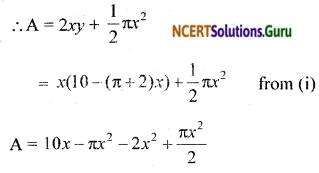iii. Differentiating A w.r.t. x,
∴ A’ = 10 – 2 πx – 4x + π x
A’ = 10 – π x – 4x
A” = – π – 4 = – (π + 4)
For maxima, A’ = 0
10 – πx – 4x = 0
x(π + 4) = 10
x = $$\frac { 10 }{ π + 4 }$$
When x = $$\frac { 10 }{ π + 4 }$$, A” = – (π + 4) < 0
∴ A is maximum when x = $$\frac { 10 }{ π + 4 }$$
2y = 10 – (π + 2)x
= 10 – (π + 2)$$\frac { 10 }{ π + 4 }$$
= $$\frac{10 \pi+40-10 \pi-20}{\pi+4}$$ = $$\frac { 20 }{ π + 4 }$$
∴ y = $$\frac { 10 }{ π + 4 }$$
∴ Length of the window = 2x = $$\frac { 20 }{ π + 4 }$$
Length of larger side of window = y = $$\frac { 10 }{ π + 4 }$$.

Question 12.
A point on the hypotenuse of a triangle is at distance a and b from the side of the tri¬angle. Show that the minimum length of the hypotenuse is $$\left(a^{\frac{2}{3}}+b^{\frac{2}{3}}\right)^{\frac{3}{2}}$$.
Solution:Let ∆ABC be right angled at B and P be a point on the hypotenuse AC at a distance of a from BC and b from AB.
∴ PQ = a and PR = b
Let ∠A = θ then ∠C = 90 – θ
From right triangles ARP and PQC, we get
AP = $$\frac { b }{ sin θ }$$ = b cosec θ
PC = $$\frac { a }{ sin(90 – θ) }$$ = $$\frac { a }{ cos θ }$$ = asec θ
Let l be the length of the hypotenuse
∴ l = AP + PC = asecθ + bcosecθ,
0 < θ < $$\frac { π }{ 2 }$$
$$\frac { dl }{ dθ }$$ = asecθ tanθ – bcosecθ cotθ
$$\frac{d^{2} l}{d \theta^{2}}$$ = a[secθ.sec²θ + tanθ.secθ tanθ] – b[cosecθ(-cosec²θ) + cot θ(-cosecθ cotθ)] = a[sec³θ + secθtan²θ] + b[cosec³θ + cosecθ cot²θ]
For minima
$$\frac { dl }{ dθ }$$ = 0 ⇒ asecθ tanθ – bcosecθ cotθ = 0
⇒ a secθ tanθ = b cosecθ cotθ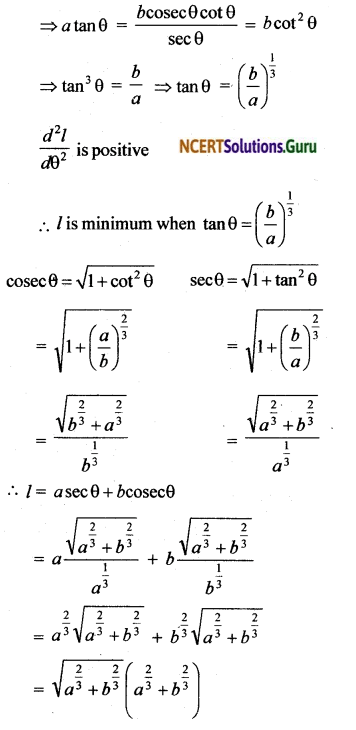Minimum length of the hypotenuse = $$\left(a^{\frac{2}{3}}+b^{\frac{2}{3}}\right)^{\frac{3}{2}}$$.Question 13.
Find the points at which the function given by f(x) = (x – 2)4 (x + 1)³ has
i. local maxima
ii. local minima
iii. point of in flexion
Solution:
f(x) = (x – 2)4 (x + 1)³
f’(x) = (x – 2)4[3(x + 1)²] + (x+ 1)³[4(x – 2)³]
= (x – 2)³(x + 1)²[3x – 6 + 4x + 4]
= (x – 2)³(x + 1)²(7x – 2)
f’(x) = 0 ⇒ (x – 2)³(x + 1)²(7x – 2) = 0
⇒ x = – 1, and x = $$\frac { 2 }{ 7 }$$ x = 2
Let us apply first derivative test.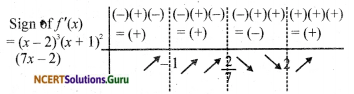i. local maxima is at x = $$\frac { 2 }{ 7 }$$
ii local minima ¡s at x = 2
iii. point of inflexion is at x = – 1

Question 14.
Find the absolute maximum and minimum values of the function f given by
f(x) = cos² x + sin x, x ∈ [0, π]
Solution:
f(x) = cos² x + sin x, x ∈ [0, π]
f'(x) = – 2cosx sinx + cosx = cosx (1 – 2sinx)
f'(x) = 0 ⇒ cosx (1 – 2sinx) = 0
⇒ cosx = 0 or sin x = $$\frac { 1 }{ 2 }$$
⇒ x = $$\frac { π }{ 2 }$$ or x = $$\frac { π }{ 6 }$$ and x = $$\frac { 5π }{ 6 }$$
∴ x = $$\frac { π }{ 6 }$$, $$\frac { π }{ 2 }$$, $$\frac { 5π }{ 6 }$$
f(0) = cos²0 + sin0 = 1² + 0 = 1Question 15.
Show that the altitude of the right circular cone of maximum volume that can be in-scribed in a sphere of radius r is $$\frac { 4r }{ 3 }$$
Solution:
Let R be the radius and A be the height of the right circular cone inscribed in a sphere of radius r.i.e., AB = h, OA = OC = r and BC = R
In right triangle OBC, OB = h – r
and OC² = OB² + BC²
i.e., r² = (h – r)² + R²
r² = h² – 2 hr + r² + R²
∴ R² = 2hr – h²
Volume of the cone V = $$\frac { 1 }{ 3 }$$ πR²h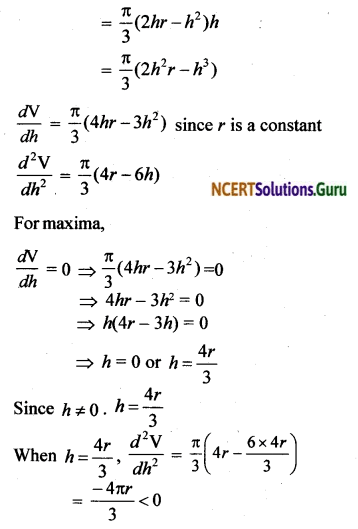∴ When h = $$\frac { 4r }{ 3 }$$, the volume is maximum.Question 16.
Let f be a function defined on [a, b] such that f'(x) > 0, for all x ∈ (a, b). Then prove that f is an increasing function on (a, b)
Solution:
Let c1, c2 ∈ [a , b\ such that c1, c2.
Since f'(x) > 0 in (a, b), f is continuous in [a, b] and differentiable in (a, b).
∴ By mean value theorem, there exists c ∈ (c1, c2) such that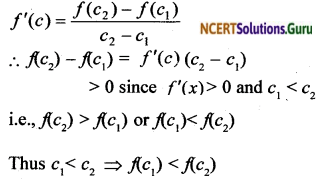Since c1 and c2 are arbitrary, we get f(x) is increasing on (a, b).

Question 17.
Show that the height of the cylinder of maxi-mum volume that can be inscribed in a sphere of radius R is $$\frac{2 \mathrm{R}}{\sqrt{3}}[/latex . Also find the maximum volume. Solution:Let y be the radius and x be the height of the cylinder.∴ V is maximum when x = [latex]\frac{2 \mathrm{R}}{\sqrt{3}}$$
The height of cylinder with maximum volume is $$\frac{2 \mathrm{R}}{\sqrt{3}}$$
∴ (1) → Maximum volume,
V = $$\frac{\pi}{4}\left(4 \mathrm{R}^{2} \cdot \frac{2 \mathrm{R}}{\sqrt{3}}-\frac{8 \mathrm{R}^{3}}{3 \sqrt{3}}\right)=\frac{4 \pi \mathrm{R}^{3}}{3 \sqrt{3}}$$

Question 18.
Show that the height of the cylinder of greatest volume which can be inscribed in a right circular cone of height h and semi vertical angle a is one-third that of the cone and the greatest volume of cylinder is $$\frac { 4 }{ 27 }$$πh³ tan²α.
Solution: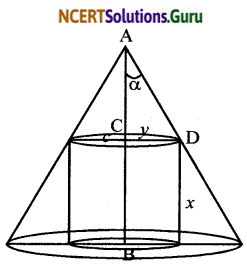Let y be the radius and x be the height of the right circular cylinder inscribed in a cone of height h and semivertical angle α.
Then AB h, CD = y, BC = x
∴ AC = h – x
From right triangle ACD, tan α = $$\frac { CD }{ AC }$$
= $$\frac { y }{ h – x }$$
⇒ y = (h – x)tan α
Volume of the cylinder V = πy²x
= π(h – x)² tan² α.x∴ V is maximum when the height of the cylinder is one third of the height of the cone.
(1) → Maximum volume,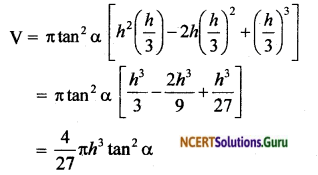Question 19.
A cylindrical tank of radius 10 m is being filled with wheat at the rate of 314 cubic metre per hour. Then the depth of the wheat is increasing at the rate of
a. 1 m³/h
b. 0.1 m³/h
c. 1.1 m³/h
d. 0.5 m³/h
Solution:
a. 1 m³/h
Let h be the height of the wheat at time t and V be the volume at that instantQuestion 20.
The slope of the tangent to the curve x = t² + 3t – 8, y = 2t² – 2t – 5 at the point (2, – 1) is
a. $$\frac { 22 }{ 7 }$$
b. $$\frac { 6 }{ 7 }$$
c. $$\frac { 7 }{ 6 }$$
d. $$\frac { – 6 }{ 7 }$$
Solution:
b. $$\frac { 6 }{ 7 }$$
x = t² + 3t – 8 and y = 2t² – 2t – 5
Put x = 2 and y = – 1, we get
t² + 3t – 8 = 2 and 2t² – 2t – 5 = – 1
i.e., t² + 3t – 10 = 0 and 2t² – 2t – 4 = 0
i.e, (t + 5) (t – 2) = 0 and 2(t – 2) (t+ 1) = 0
i.e., t = -5 or t = 2 and t = 2 or t = – 1
Hence t = 2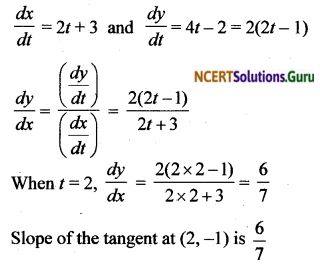Question 21.
The line y = mx + 1 is a tangent to the curve y² = 4x if the value of m is
a. 1
b. 2
c. 3
d. $$\frac { 1 }{ 2 }$$
Solution:
y² = 4x
Differentiating w.r.t x,
2y$$\frac { dy }{ dx }$$ = 4 ⇒ $$\frac { dy }{ dx }$$ = $$\frac { 2 }{ y }$$
∴ Slope of the tangent, m = $$\frac { 2 }{ y }$$
∴ y = mx + 1 becomes y = $$\frac { 2 }{ y }$$ x + 1
y² = 2x + y
i.e., y² = $$\frac { y² }{ 2 }$$ + y since y² = 4x
$$\frac { y² }{ 2 }$$ = y ⇒ y² = 2y
⇒ y(y – 2) = 0
y ≠ 0 since slope = $$\frac { 2 }{ y }$$
∴ y = 2
∴ slope m = $$\frac { 2 }{ y }$$ = $$\frac { 2 }{ 2 }$$ = 1

Question 22.
The normal at the point (1,1) on the curve 2y + x² – 3 is
a. x + y = 0
b. x – y = 0
c. x + y + 1 = 0
d. x – y = 0
Solution:
b. x – y = 0
2y + x² = 3
Differentiating w.r.t x,
2$$\frac { dy }{ dx }$$ + 2x = 0
$$\frac { dy }{ dx }$$ = – x
Slope of the tangent at (1, 1)
= $$\frac { dy }{ dx }$$ at (1, 1) = – 1
∴ Slope of the normal at (1, 1) = $$\frac { – 1 }{ – 1 }$$ = 1
Equation of the normal at (1, 1) is
y – 1 = 1(x – 1)
⇒ x – y = 0Question 23.
The normal to the curve x² = 4y passing through (1, 2) is
a. x + y = 3
b. x – y = 3
c. x + y = 1
d. x – y = 1
Solution:
a. x + y = 3
x² = Ay
Differentiating w.r.t. x,
2x = 4$$\frac { dy }{ dx }$$
∴$$\frac { dy }{ dx }$$ = $$\frac { x }{ 2 }$$
Slope of the tangent = $$\frac { x }{ 2 }$$
∴ Slope of the normal = $$\frac { – 2 }{ x }$$
Let (x1, y1) be a point on the curve
∴ Slope of normal at (x1, y1) = $$\frac{-2}{x_{1}}$$
Equation of the normal at (x1, y1) is
y – y1 = $$\frac{-2}{x_{1}}$$(x – x1)
The normal passes through the point (1, 2)
∴ (2 – y1) = $$\frac{-2}{x_{1}}$$(1 – x1)
Equation of the normal at(x1, y1) is
y – y1 = $$\frac{-2}{x_{1}}$$(1 – x1)
2 – y1 = $$\frac{2}{x_{1}}$$ + 2
∴ y1 = $$\frac{2}{x_{1}}$$
Since (x1, y1) is a point on x² = 4y, we get
x1² = 4y1
i.e., x1³ = $$\frac{4 \times 2}{x_{1}}$$, sjnce y1 = $$\frac{2}{x_{1}}$$
x1² = 8 ⇒ x, = 2
When x1 = 2, y1 = $$\frac { 2 }{ 2 }$$ = 1
∴ Equation of the normal at (2, 1) is
y – 1 = $$\frac { – 2 }{ 2 }$$(x – 2)
y – 1 = – (x – 2)
i.e., x + y = 3

Question 24
The points on the curve 9y² = x³, where the normal to the curve makes equal intercepts with the axes are
a. $$\left(4, \pm \frac{8}{3}\right)$$
b. $$\left(4, \pm \frac{-8}{3}\right)$$
c. $$\left(4, \pm \frac{3}{8}\right)$$
d. $$\left(\pm 4, \frac{3}{8}\right)$$
Solution:
9y² = x³
Let (x1, y1) be the point at which the nor¬mal make equal intercepts on the coordinate axes.
Differentiating 9y³ = x³ w.r.t. x, we get
18y$$\frac { dy }{ dx }$$ = 3x²
$$\frac { dy }{ dx }$$ = $$\frac{x^{2}}{6 y}$$
Slope of the tangent at (x1, y1) = $$\frac{x_{1}^{2}}{6 y_{1}}$$
∴ Slope of normal at (x1, y1) = $$\frac{-6 y_{1}}{x_{1}^{2}}$$
Equation of the normal at (x1, y1) is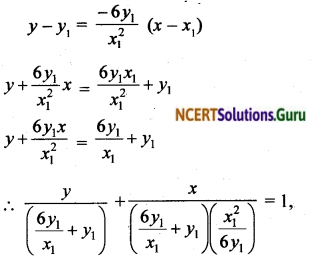which is in the intercept form.
Since the intercepts of the normal are equal,we get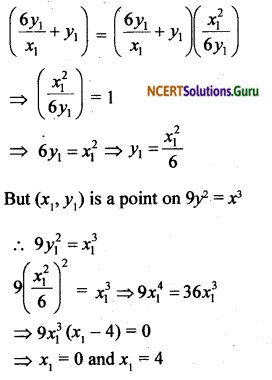But x1 ≠ 0 since x1 = 0 makes the intercepts meaningless.
∴ x1 = 4
When x1, 9y²1 = x³1 becomes
9y²1 = 4³ = 64
1 = $$\frac { 64 }{ 9 }$$ ⇒ y1 = ≠ $$\frac { 8 }{ 3 }$$
∴ The required points are (4, $$\frac { 8 }{ 3 }$$)

error: Content is protected !!